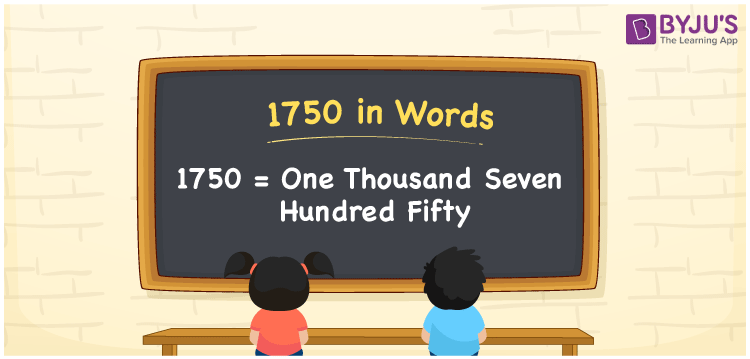# 1750 in Words

1750 in words is written as One thousand seven hundred fifty. If you got a coupon worth Rs. 1750, you can convey the same to others as “I got a coupon worth One thousand seven hundred fifty rupees”. Similarly, we use the word form of the cardinal number 1750 in many discussions.

 1750 in words One thousand seven hundred fifty One thousand seven hundred fifty in Numbers 1750

## 1750 in English Words

Using the English alphabet, we can write numbers in words. So, we can read 1750 in words as “One thousand seven hundred fifty.## How to Write 1750 in Words?

We can convert the number 1750 into words using a four-column place value chart since it has four digits. Thus, we need to write the place value for each of these four digits as shown below.

 Thousands Hundreds Tens Ones 1 7 5 0

Here, ones = 0, tens = 5, hundreds = 7, and thousands = 1.

Thus, we can write the expanded form as:

1 × Thousand + 7 × Hundred + 5 × Ten + 0 × One

= 1 × 1000 + 7 × 100 + 5 × 10 + 0 × 1

= 1000 + 700 + 50

= One thousand + Seven hundred + Fifty

= One thousand seven hundred fifty

Hence, 1750 in words = One thousand seven hundred fifty.

1750 is a natural number that precedes 1751 and succeeds 1749.

1750 in words – One thousand seven hundred fifty

Is 1750 an odd number? – No

Is 1750 an even number? – Yes

Is 1750 a prime number? – No

Is 1750 a composite number? – Yes

Is 1750 a perfect square number? – No

Is 1750 a perfect cube number? – No

## Frequently Asked Questions on 1750 in Words

Q1

### How do you write 1750 in words?

We can write 1750 in English words as One thousand seven hundred fifty.
Q2

### How to write 1750 rupees in words on a cheque?

On a cheque, we should write 1750 rupees in words as One thousand seven hundred fifty rupees only.
Q3

### How do you convert 1750 to word form?

We can convert the number 1750 to word form using the place values of digits of that number. This can be done as 1750 = 1000 + 700 + 50 = One thousand + Seven hundred + Fifty = One thousand seven hundred fifty.